// This prints the left floatting menu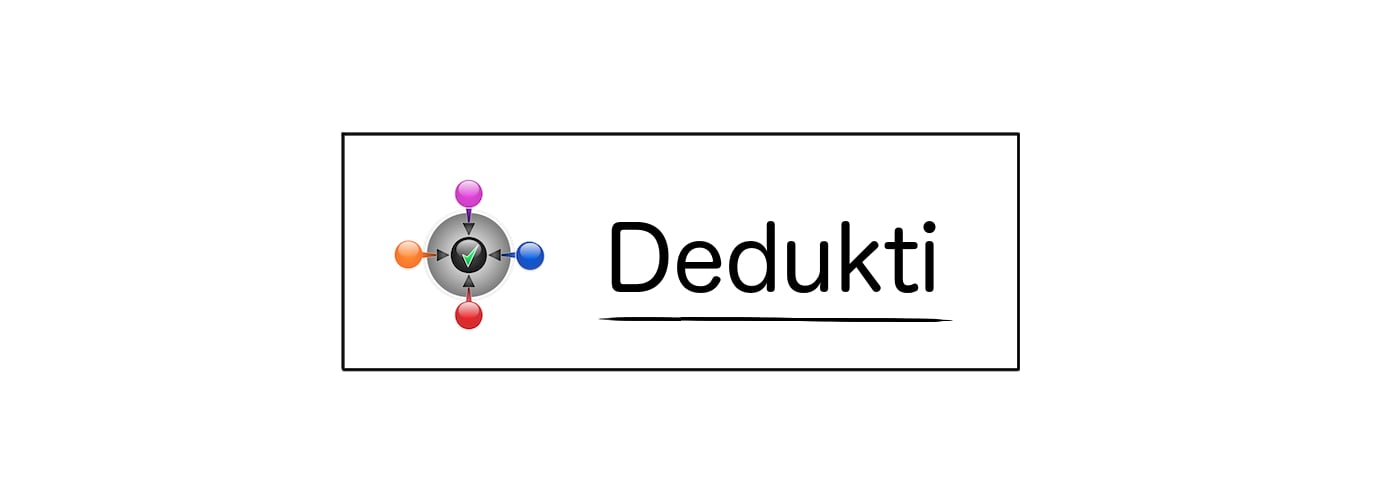### Theoremlogic.rewrite_r

Statement

∀ x P, P x ⇒ ∀ y, y = x ⇒ P y

Main Dependencies
Theory
constant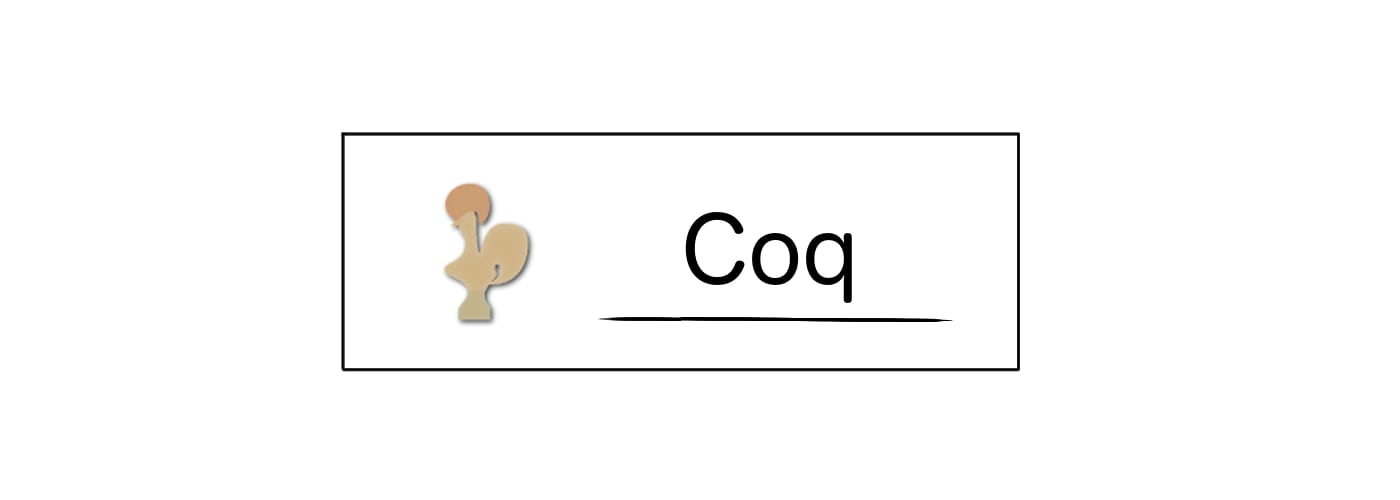Statement

Theorem rewrite_r : forall A, forall (x:A), forall (P:(A -> Prop)), (P x) -> forall (y:A), (eq (A) y x) -> P y.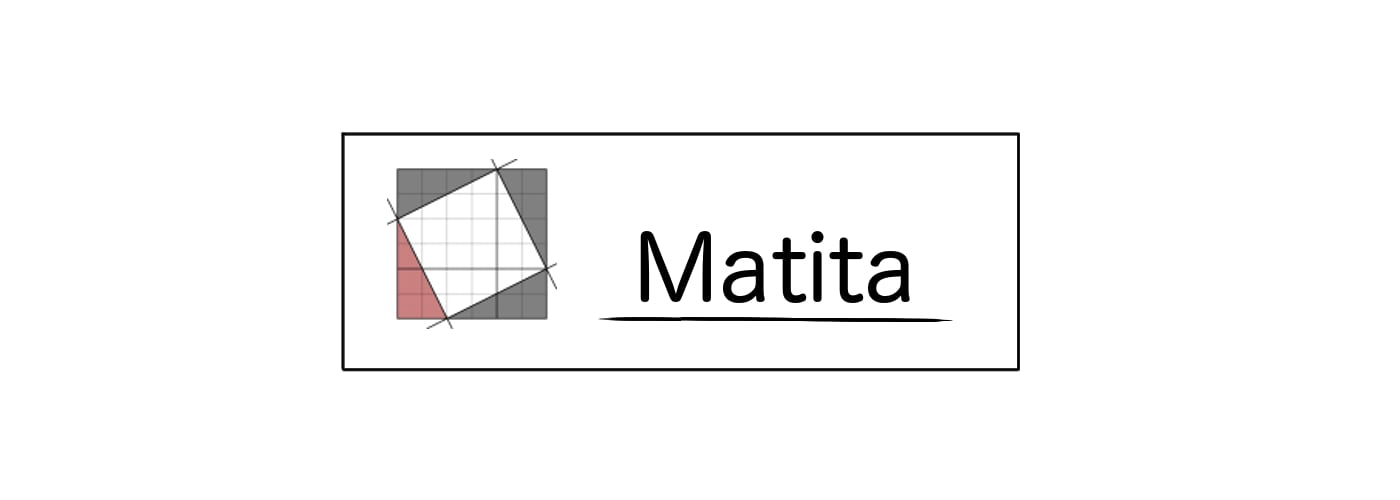Statement

theorem rewrite_r : \forall A. \forall (x:A). \forall (P:A -> Prop). (P x) -> \forall (y:A). ((eq) (A) y x) -> P y.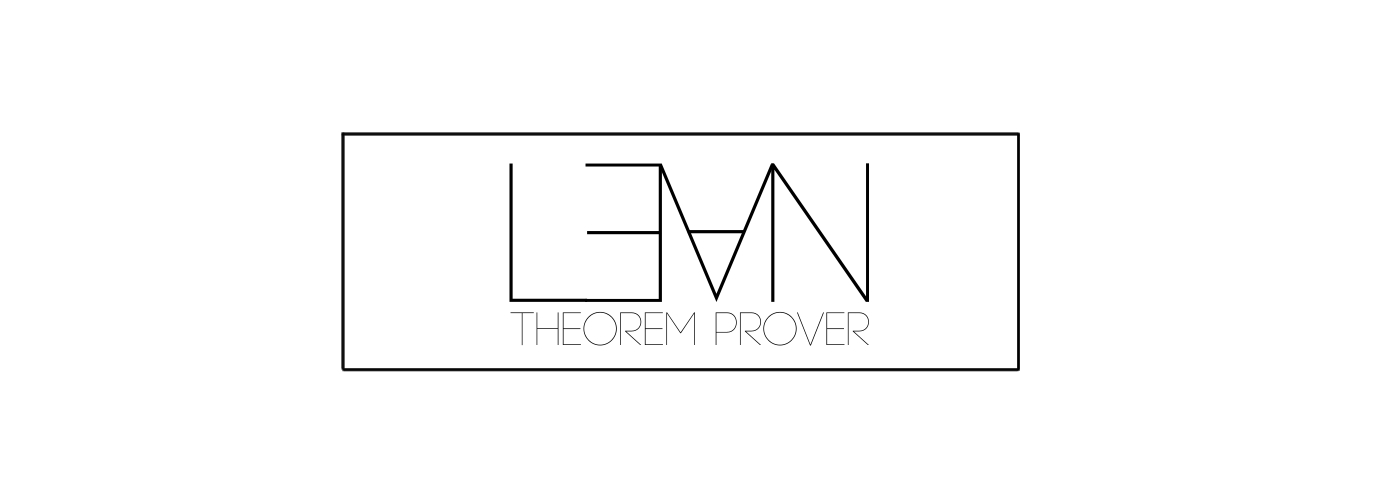Statement

theorem rewrite_r : forall (A : Type) , forall (x:A) , forall (P:(A) -> Prop) , ((P) (x)) -> forall (y:A) , ((((logic.eq_) (A)) (y)) (x)) -> (P) (y).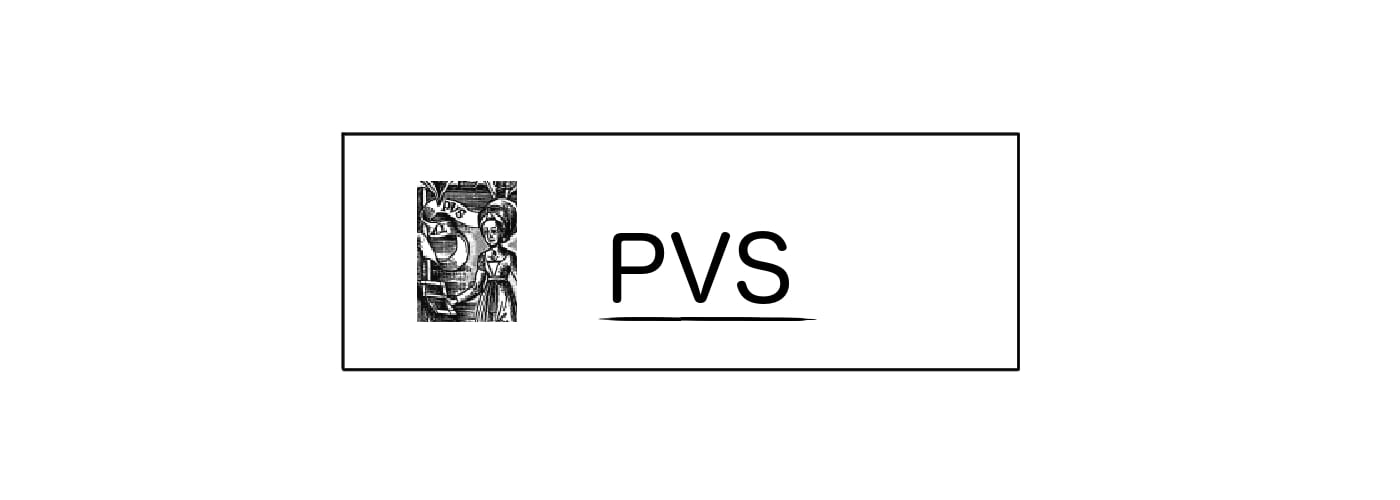Statement

rewrite_r [A:TYPE+] : LEMMA (FORALL(x:A):(FORALL(P:[A -> bool]):(P(x) => (FORALL(y:A):(logic_sttfa.eq[A](y)(x) => P(y))))))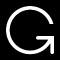Printing for OpenTheory is not working at the moment.